# Class 9 Maths Extra and Important Questions for Probability

Given below are the Class 9 Maths Extra Questions for Probability
a. Concepts questions
b. Calculation problems
c. Multiple choice questions
e. Fill in the blanks

Question 1
Twenty four people had a blood test and the results are shown below.
A , B , B , AB , AB , B , O , O , AB , O , B , A
AB , A , O , O , AB , B , O , A , AB , O , B , A
(a) Construct a frequency distribution for the data.
(b) If a person is selected randomly from the group of twenty four people, what is the probability that his/her blood type is not O?
Solution
(a)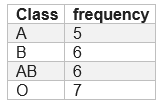(b)
$1 - \frac {7}{24} = \frac {17}{24} = 0.71$ (rounded to 2 decimal places)

Question 2
Over the past 100 working days, the number of defective bulbs produced by a machine is given in the following table: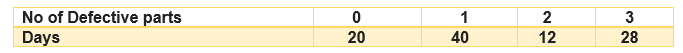a. The probability that tomorrow output will be defect free is .2
b. The probability that tomorrow output will have at least 1 defect is .8
c. The probability that tomorrow output will have more then  2 defect is  .30
d. The probability that tomorrow output will have 3 defects is .28
Solution
a. True , P= $\frac {20}{100}=.2$
b. True  , P= $\frac {(40+12+28)}{100}=.8$
c. False ,P= $\frac {28}{100}=.28$
d. True. P= $\frac {28}{100}=.28$

## Multiple choice Questions

Question 3
The probability of the events lies between
a. -1 ≤ p ≤ 1
b. 0 ≤ p ≤ 1
c. -1 ≤ p ≤ 0
d. -1 < p ≤ 1
Solution (b)

Question 4
Twelve bags of wheat flour, each marked 5 kg, actually contained the following weights of flour (in kg):
4.97 5.05 5.08 5.03 5.00 5.06 5.08 4.98 5.04 5.07 5.00 5.12
Find the probability that any of these bags chosen at random contains more than 5 kg
of flour
a. $\frac {1}{12}$
b. $\frac {7}{12}$
c. $\frac {2}{3}$
d. None of these
Solution (c)
No of bags having weight more than 5 kg=8
Total =12
So $P= \frac {8}{12} = \frac {2}{3}$

Question 5
A company selected 4000 households at random and surveyed them to find out a relationship between income level and the number of mobile sets in a home. The information so obtained is listed in the following table: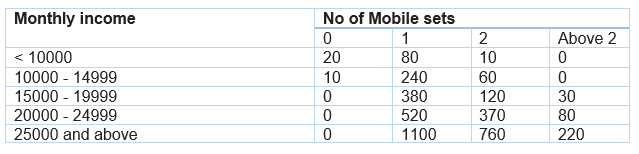Find the probability of a household earning Rs 10000 – Rs 14999 per year and having exactly one mobile set
a. $.06$
b. $.08$
c. $.04$
d. None of these
Solution (a)
Around 240 household are there satisfying the condition
So $P= \frac {240}{4000}=.06$

Question 6
In the above question, Find the probability of a household earning more than 25000 per year and having exactly 2 mobile set
a. $.2$
b. $.19$
c. $.12$
d. $.3$
Solution (b)
Around 760 household are there satisfying the condition
So $P= \frac {760}{4000}=.19$

Question 7
In the above question, find the probability of a household earning more than 25000 per year and having 2 or more mobile set
a. $.245$
b. $.3$
c. $.1$
d. None of these
Solution (a)
Around 980 household are there satisfying the condition
So $P= \frac {980}{4000}=.245$

Question 8
In the above question, Find the probability of a household having no mobile set at all?
a. $\frac {3}{400}$
b. $\frac {1}{400}$
c. $\frac {1}{200}$
d. None of these
Solution (a)
Around 30 household are there satisfying the condition
So $P= \frac {30}{4000}= \frac {3}{400}$

Question 9
In the above question, Find the probability of a household having 3 mobile set and having income less than 10000
a. .1
b. 0
c. .24
d. None of these
Solution (b)
As no household exists  like than, So probability is 0

Question 10.
A parent has collected data of number of school based on the monthly fees, so that he can choose the school for admission of his child. Data is as under: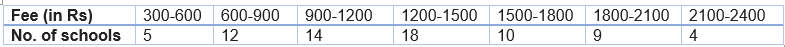If a school is selected at random, find the probability that the school is having –
1. minimum fees
2. maximum fees
3. fees less than 2900
4. fees at least Rs 1500

Question 11.
A survey of 2000 people of different age groups was conduct to find out their preference n watching different types of movies.
Type I – family
Type II – comedy & family
Type III – romantic, comedy & family
Type IV – action, romantic, comedy & family
The data recorded was as follows –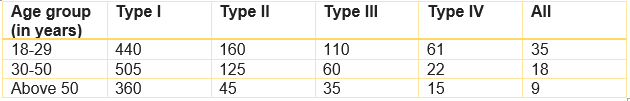Find the probability that person chosen at random is-
1. in 18-29 years of age and likes type II movies
2. above 50 years of age and likes all types of movies
3. in 30-50 years and likes type I movies.

Question 12.
An insurance company selected 2000 drivers at random in a particular city to find a relationship between age and accidents. The data obtained are given in following table-
Age groups of               no. of accidents
Drivers (in years)            0          1          2           3          more than 3
18-29                            440       160      110        61            35
30-50                            505       125        60        21           18
above 50                       360         45        35        15           10
Find the probability:-
1. being 18-29 years of age and having exactly 3 accidents.
2. being 30-50 years of age and having one or more accidents in a year.
3. having no accidents in 1 year.
4. which value would you like to remember from data?

Question 13.
Find the probability that a leap year, selected at random will have 53 Sundays
A normal year(365 days) has 52 Mondays, 52 Tuesdays, 52 Wednesdays, 52 Thursdays, 52 Fridays, 52 Saturdays and 52 Sundays + 1 day( 7 X 52 +1) that could be anything depending upon the year under consideration. In addition to this, a leap year(366) has an extra day which might be a Monday or Tuesday or Wednesday...or Sunday.

So Now our question is reduced to finding the consecutive pairs of the year where one of them is a Sunday

Our sample space is S : {Monday-Tuesday, Tuesday-Wednesday, Wednesday-Thursday,..., Sunday-Monday}
Number of elements in S = n(S) = 7
Now Saturday-Sunday and Sunday-Monday satisfies our requirement(2)
probability of occurrence of 53 Sundays in leap = $\frac {2}{7}$

Question 14.
A coin is tossed 15 times and observed that 11 times head comes up. Find the probability that a tail comes up
no. of tails = 15-11
probability of tails = $\frac {4}{15}$

## Summary

This Probability class 9 extra questions is prepared keeping in mind the latest syllabus of CBSE . This has been designed in a way to improve the academic performance of the students. If you find mistakes , please do provide the feedback on the mail.

• Notes
• NCERT Solutions & Assignments